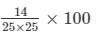# RD Sharma Solutions - Chapter 12 - Percentage (Part - 1), Class 8, Maths Notes | Study RD Sharma Solutions for Class 8 Mathematics - Class 8

## Class 8: RD Sharma Solutions - Chapter 12 - Percentage (Part - 1), Class 8, Maths Notes | Study RD Sharma Solutions for Class 8 Mathematics - Class 8

The document RD Sharma Solutions - Chapter 12 - Percentage (Part - 1), Class 8, Maths Notes | Study RD Sharma Solutions for Class 8 Mathematics - Class 8 is a part of the Class 8 Course RD Sharma Solutions for Class 8 Mathematics.
All you need of Class 8 at this link: Class 8

PAGE NO 12.2:

Question 1:

Write each of the following as percent:
(i) 7/25
(ii) 14/625
(iii) 5858
(iv) 0.8
(v) 0.005
(vi) 3 : 25
(vii) 11 : 80
(viii) 111 : 125
(ix) 13 : 75
(x) 15 : 16
(xi) 0.18
(xii) 7/125

(i) 7/25 = 7/25 × 100

= 700/25 = 28%

(ii) 14/625 == 1400/625

= 2.24%

(iii) 5/8 = 0.625 × 100

= 62.5%

(iv) 0.8 = 8/10 × 100

= 80%

(v) 0.005 = 0.005 × 100

= 0.5%

(vi) 3:25 = 3/25 × 100

= 300/25

= 12%

(vii) 11:80 = 11/80 × 100

= 1100/80

= 13.75%

(viii) 111:125 = 111/125

= 0.888 × 100

= 88.8%

(ix) 13:75 = 13/75

= 0.1733 × 100

= 17.33%

(x) 15:16 = 1516 = 0.9375 × 100

= 93.75%

(xi) 0.18 = 18/100

= 18/100 × 100

= 18%

(xii) 7/125 = 0.056

= 0.056 × 100

= 5.6%

PAGE NO 12.2:

Question 2:

Convert the following percentages to fractions and ratios:
(i) 25%
(ii) 2.5%
(iii) 0.25%
(iv) 0.3%
(v) 125%

(i) 25% = 25/100

= 5/20

= 1/4

∴ Ratio = 1:4

(ii) 2.5% = 2.5/100

= 25/1000 = 5/200

= 1/40

∴ Ratio = 1:40

(iii) 0.25% = 0.25/100

= 25/10000 = 5/2000

= 1/400

∴ Ratio = 1:400

(iv) 0.3% = 0.3/100

= 3/1000

∴ Ratio = 3:1000

(v) 125% = 125/100

= 5/4

∴ Ratio = 5:4

PAGE NO 12.2:

Question 3:

Express the following as decimal fractions:
(i) 27%
(ii) 6.3%
(iii) 32%
(iv) 0.25%
(v) 7.5%
(vi) 18%

(i) 27% = 27/100

= 0.27

(ii) 6.3% = 6.3/100

= 0.063

(iii) 32% = 32/100

= 0.32

(iv) 0.25% = 0.25/100

= 0.0025

(v) 7.5% = 7.5/100

= 0.075

(vi) 18% = 1/8 × 1/100

= 1/800

= 0.00125

The document RD Sharma Solutions - Chapter 12 - Percentage (Part - 1), Class 8, Maths Notes | Study RD Sharma Solutions for Class 8 Mathematics - Class 8 is a part of the Class 8 Course RD Sharma Solutions for Class 8 Mathematics.
All you need of Class 8 at this link: Class 8Use Code STAYHOME200 and get INR 200 additional OFF

## RD Sharma Solutions for Class 8 Mathematics

88 docs

Track your progress, build streaks, highlight & save important lessons and more!

,

,

,

,

,

,

,

,

,

,

,

,

,

,

,

,

,

,

,

,

,

,

,

,

,

,

,

;